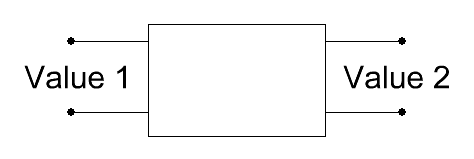audio with realistic timbre

# Berekenen van versterking (gain) en demping (loss)

Enter two values and press the desired calculate bar
 Input:voltagepower input value 1 output value 2 level change dB

For now, we deal only with 'voltage' amplification (gain) and damping (loss).V2 >V 1 = amplification, dB value is positive and V 2< V 1 = damping, dB value is negative.
V2 / V1 means the ratio. The amplification or the damping in dB is:
L = 20 × log (voltage ratio V 2 / V 1)         V 1 is the reference.

 3 dB ≡ 1.414 fold the voltage - 3 dB ≡ damping to the value 0.707 6 dB ≡ 2 fold the voltage - 6 dB ≡ damping to the value 0.5 10 dB ≡ 3.162 fold the voltage -10 dB ≡ damping to the value 0.316 20 dB ≡ 10 fold the voltage -20 dB ≡ damping to the value 0.1

Using voltage we get: Level in dB: L = 20 × log (voltage ratio)

+6 dB = two fold the voltage
+12 dB = four fold the voltage
+20 dB = ten fold the voltage
+40 dB = hundred fold the voltage

Using power we get: Level in dB: L = 10 × log (power ratio)

+3 dB = two fold the power
+6 dB = four fold the power
+10 dB = ten fold the power
+20 dB = hundred fold the power

Bron: Sengpielaudio.com

*

_______________